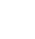# Tag Archives: random number

## Random number generator in Excel

To generate a ‘Random’ set of numbers in Excel you just need to use the Randbetween formula as follows: =Randbetween(1,5000) This will put a random number between 1 and 5000 in the cell where the formula is. Drag this down to as many cells as you like.